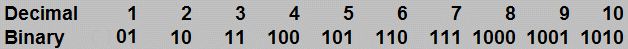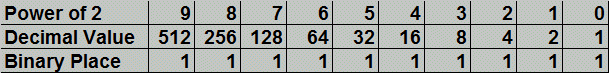Parents and Teachers: Support Ducksters by following us onor.

# Kids Math

## Binary Numbers

Summary

The binary number system is a base-2 number system. This means it only has two numbers: 0 and 1. The number system that we normally use is the decimal number system. It has 10 numbers: 0-9.

Why use binary numbers?

Binary numbers are very useful in electronics and computer systems. Digital electronics can easily work with a sort of "on" or "off" system where "on" is a 1 and "off" is a zero. Often times the 1 is a "high" voltage, while the 0 is a "low" voltage or ground.

How do binary numbers work?

Binary numbers only use the numbers 1 and 0. In a binary number each "place" represents a power of 2. For example:

1 = 20 = 1
10 = 21 = 2
100 = 22 = 4
1000 = 23 = 8
10000 = 24 = 16

Converting from Binary to Decimal

If you want to convert a number from binary to decimal, you can add up the "places" that we showed above. Each place that has a "1" represents a power of 2, starting with the 0s place.

Examples:

101 binary = 4 + 0 + 1 = 5 decimal
11110 binary = 16 + 8 + 4 + 2 + 0 = 30 decimal
10001 binary = 16 + 0 + 0 + 0 + 1 = 17 decimal

Converting from Decimal to Binary

Converting a decimal number to a binary number can be more difficult. It helps if you know the powers of two (1, 2, 4, 8, 16, 32, 64, 128, 256, …).
• First subtract the largest power of two possible from the number you are converting.
• Then put a "1" in that place of the binary number.
• Next, you subtract the next biggest power of two possible from the remainder. You put a 1 in that position.
• You keep repeating the above until there is no remainder left.
• All the places without a "1" get a "0".
Example:

What is 27 decimal in binary?

1. What is the largest power of 2 that is less than or equal to 27? That is 16. So subtract 16 from 27. 27 - 16 = 11
2. Put a 1 in the 16's place. That is 24, which is the 5th place because it starts with the 0's place. So we have 1xxxx so far.
3. Now do the same for the remainder, 11. The largest power of two number we can subtract from 11 is 23, or 8. So, 11 - 8 = 3.
4. Put a 1 in the 8's place. Now we have 11xxx.
5. Next is to subtract 21, or 2 which is 2 -1 = 1.
6. 11x1x
7. Lastly is 1-1 = 0.
8. 11x11
9. Put zero's in the places without 1's and we get the answer = 11011.

Other examples:

14 = 8 + 4 + 2 + 0 = 1110
21 = 16 + 0 + 4 + 0 + 1 = 10101
44 = 32 + 0 + 8 + 4 + 0 + 0 = 101100

First 10 NumbersBinary Position Values in Decimal (powers of 2)Back to Kids Math

Back to Kids StudyFollow us onor